# Baby Monitoring App

A monitoring system that records temperature and motion of a baby while sleeping. Notifications sent to phone and secondary photon.

IntermediateShowcase (no instructions)2 hours816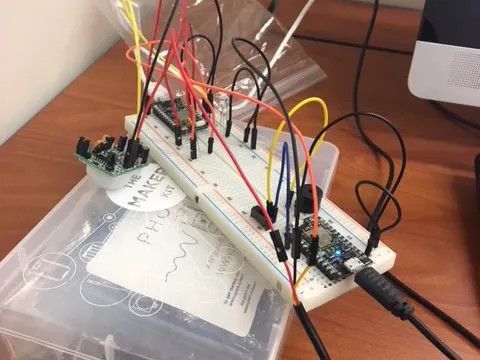## Things used in this project

### Hardware componentsParticle Photon
×2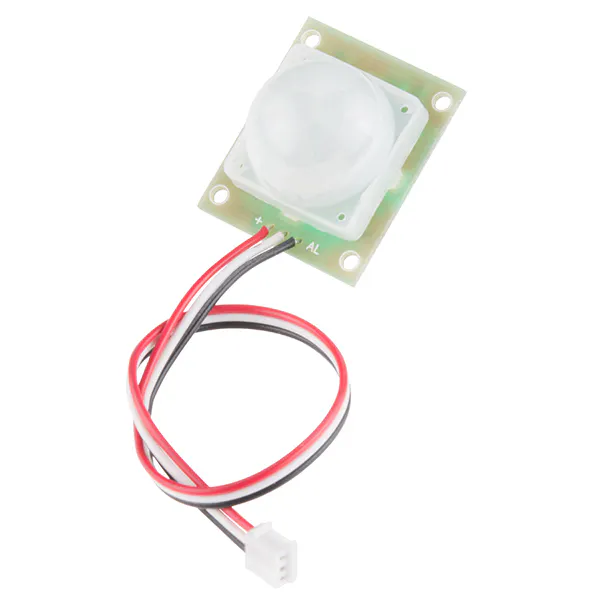PIR Motion Sensor (generic)
×1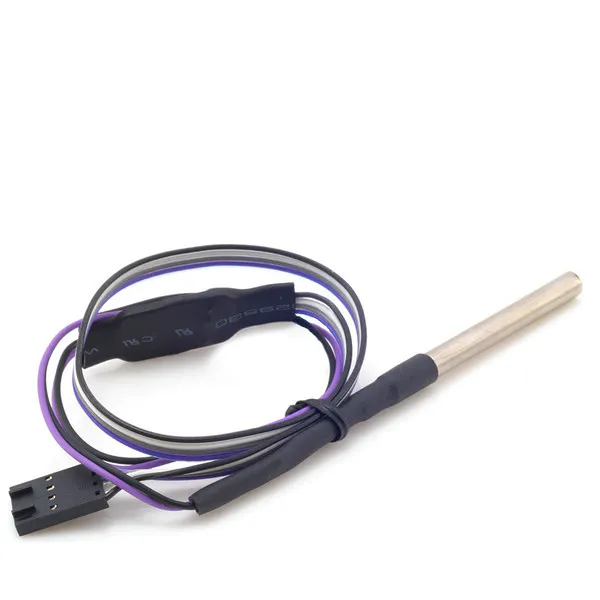Modulo Temperature Probe
×1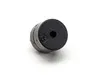Buzzer
×1Jumper wires (generic)
×1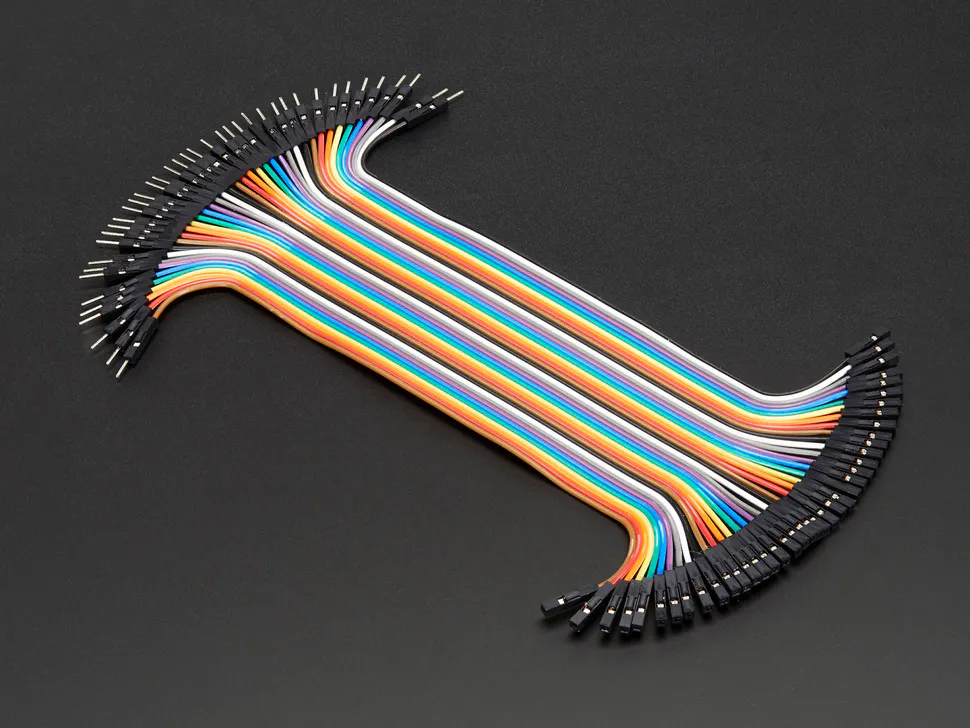Male/Female Jumper Wires
×1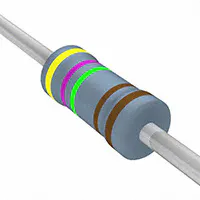Resistor 4.75k ohm
×1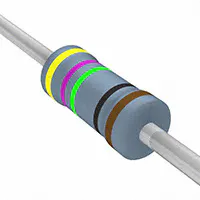Resistor 475 ohm
×1LED (generic)
×1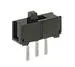Slide Switch
×1

### Software apps and online servicesBlynk

## Schematics

### Diagram of Photon 1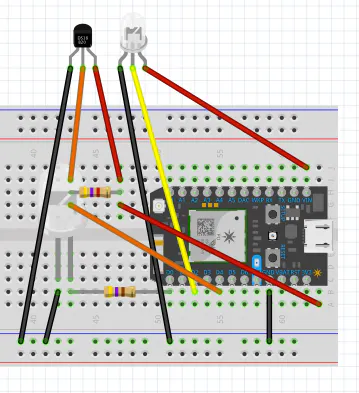### Diagram of Photon 2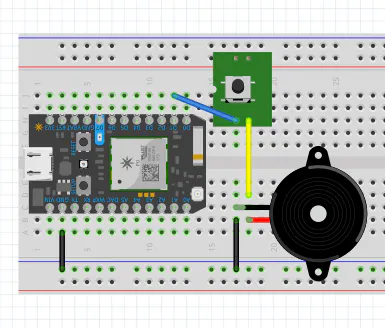## Code

### PIR/Temperature Sensor Photon

C/C++
Tests and records information on motion and temperature to determine if a baby is "safe" while sleeping
```// This #include statement was automatically added by the Particle IDE.
#include <OneWire.h>

// This #include statement was automatically added by the Particle IDE.
#include <blynk.h>

char auth[] = "0d3006572ae04fbb97828892e60e1910";
OneWire ds = OneWire(D4);  // 1-wire signal on pin D4

int ledPin = D0;                 // choose the pin for the LED
int inputPin = D2;               // choose the PIR sensor pin
bool available;                  // status of conference room
int motionCounter = 0;           // variable to count motion events

Timer timer(30000, determineMotion); // software timer to check every 30s

int pirState;
int high = 1;
int low = 0;
float lastTemp;

void setup() {
Serial.begin(9600);
delay(5000); // Allow board to settle
Blynk.begin(auth);

pinMode(ledPin, OUTPUT);       // set LED as output
pinMode(inputPin, INPUT);      // set sensor as input

timer.start(); // start the determineMotion timer
}

void loop() {
Blynk.run();
// determineInput();
if (digitalRead(inputPin) == HIGH) {  // check if the input is HIGH
digitalWrite(ledPin, HIGH);         // turn LED ON if high
Particle.publish("TorchWoodMotion","Motion Detected");
Blynk.virtualWrite(V5, high);
motionCounter++;                    // increment motion counter
} else {
digitalWrite(ledPin, LOW);          // turn LED OFF if no input
Particle.publish("TorchWoodMotion","No Motion");
Blynk.virtualWrite(V5, low);
Blynk.notify("No Motion Detected");
}
delay(500);
determineMotion();
tempdata();
}

void determineMotion() {    // this function determines if there's motion
if(motionCounter < 2) { // if very little motion was detected

if(available == false) { // only publish if the status changed
Particle.publish("TorchWoodMotion","Motion Detected");
}
available = true; // set the status to available
} else if (motionCounter >= 2) {
pirState = low;
if(available == true) { // only publish if the status changed
Particle.publish("TorchWoodMotion","No Motion");
}
available = false; // set the status to in use
}
motionCounter = 0; // reset motion counter
}

void tempdata(void) {
byte i;
byte present = 0;
byte type_s;
byte data;
float celsius, fahrenheit;

Serial.println();
ds.reset_search();
delay(250);
return;
}

// The order is changed a bit in this example
// first the returned address is printed

Serial.print("ROM =");
for( i = 0; i < 8; i++) {
Serial.write(' ');
}

// second the CRC is checked, on fail,

Serial.println("CRC is not valid!");
return;
}
Serial.println();

// we have a good address at this point
// what kind of chip do we have?
// we will set a type_s value for known types or just return

// the first ROM byte indicates which chip
case 0x10:
Serial.println("  Chip = DS1820/DS18S20");
type_s = 1;
break;
case 0x28:
Serial.println("  Chip = DS18B20");
type_s = 0;
break;
case 0x22:
Serial.println("  Chip = DS1822");
type_s = 0;
break;
case 0x26:
Serial.println("  Chip = DS2438");
type_s = 2;
break;
default:
Serial.println("Unknown device type.");
return;
}

// this device has temp so let's read it

ds.reset();               // first clear the 1-wire bus
ds.select(addr);          // now select the device we just found
// ds.write(0x44, 1);     // tell it to start a conversion, with parasite power on at the end
ds.write(0x44, 0);        // or start conversion in powered mode (bus finishes low)

// just wait a second while the conversion takes place
// different chips have different conversion times, check the specs, 1 sec is worse case + 250ms
// you could also communicate with other devices if you like but you would need

delay(1000);     // maybe 750ms is enough, maybe not, wait 1 sec for conversion

// we might do a ds.depower() (parasite) here, but the reset will take care of it.

// first make sure current values are in the scratch pad

present = ds.reset();
ds.write(0xB8,0);         // Recall Memory 0
ds.write(0x00,0);         // Recall Memory 0

present = ds.reset();
if (type_s == 2) {
ds.write(0x00,0);       // The DS2438 needs a page# to read
}

// transfer and print the values

Serial.print("  Data = ");
Serial.print(present, HEX);
Serial.print(" ");
for ( i = 0; i < 9; i++) {           // we need 9 bytes
Serial.print(data[i], HEX);
Serial.print(" ");
}
Serial.print(" CRC=");
Serial.print(OneWire::crc8(data, 8), HEX);
Serial.println();

// Convert the data to actual temperature
// because the result is a 16 bit signed integer, it should
// be stored to an "int16_t" type, which is always 16 bits
// even when compiled on a 32 bit processor.
int16_t raw = (data << 8) | data;
if (type_s == 2) raw = (data << 8) | data;
byte cfg = (data & 0x60);

switch (type_s) {
case 1:
raw = raw << 3; // 9 bit resolution default
if (data == 0x10) {
// "count remain" gives full 12 bit resolution
raw = (raw & 0xFFF0) + 12 - data;
}
celsius = (float)raw * 0.0625;
break;
case 0:
// at lower res, the low bits are undefined, so let's zero them
if (cfg == 0x00) raw = raw & ~7;  // 9 bit resolution, 93.75 ms
if (cfg == 0x20) raw = raw & ~3; // 10 bit res, 187.5 ms
if (cfg == 0x40) raw = raw & ~1; // 11 bit res, 375 ms
// default is 12 bit resolution, 750 ms conversion time
celsius = (float)raw * 0.0625;
break;

case 2:
data = (data >> 3) & 0x1f;
if (data > 127) {
celsius = (float)data - ((float)data * .03125);
}else{
celsius = (float)data + ((float)data * .03125);
}
}

// remove random errors
if((((celsius <= 0 && celsius > -1) && lastTemp > 5)) || celsius > 125) {
celsius = lastTemp;
}

fahrenheit = celsius * 1.8 + 32.0;
lastTemp = celsius;
Serial.print("  Temperature = ");
Serial.print(celsius);
Serial.print(" Celsius, ");
Serial.print(fahrenheit);
Serial.println(" Fahrenheit");

// now that we have the readings, we can publish them to the cloud
String temperature = String(fahrenheit); // store temp in "temperature" string
Particle.publish("Temperature", temperature); // publish to cloud
Blynk.virtualWrite(V6, fahrenheit);
if (fahrenheit > 100 || fahrenheit < 90)
{
if (fahrenheit > 100)
Blynk.notify("Too Hot");
if (fahrenheit < 90)
Blynk.notify("Too Cold");
}
delay(3000); // 5 second delay
}
```

### Buzzer Motion Alarm

C/C++
Takes recorded data from PIR where photon runs and creates a notification when certain parameters are met.
```// This #include statement was automatically added by the Particle IDE.
#include <blynk.h>

// This #include statement was automatically added by the Particle IDE.

#define BLYNK_PRINT Serial
char auth[] = "0d3006572ae04fbb97828892e60e1910";

int buzzerPin = D2;              // choose the input pin (for PIR sensor)
//int ledPin = D4;                // LED Pin
//int pirState = LOW;             // we start, assuming no motion detected
//int val = 0;                    // variable for reading the pin status

// wait for the thingy to calibrate

void setup() {
Serial.begin(9600);
delay(5000); // Allow board to settle

Blynk.begin(auth);
Particle.subscribe("TorchWoodMotion", RoseTyler);
pinMode(buzzerPin, OUTPUT);
// pinMode(inputPin, INPUT);     // declare sensor as input

}

void loop() {
Blynk.run();

}

void RoseTyler(const char *event, const char *data)
{
if (strcmp(data,"No Motion")==0) {
// if your buddy's beam is intact, then turn your board LED off
Particle.publish("TorchWoodBuzzer", "Buzzer ON");
digitalWrite(buzzerPin,HIGH);
delay(500);
digitalWrite(buzzerPin,LOW);

}
else if (strcmp(data,"Motion Detected")==0) {
// if your buddy's beam is broken, turn your board LED on
Particle.publish("TorchWoodBuzzer", "Buzzer OFF");
digitalWrite(buzzerPin,LOW);
}

}
```